# Mathemagical Properties of 1105

1105 is the magic sum of a 13 × 13 magic square. Why?
Because 13×13 = 169 and 169×170÷2÷13 = 13×85 = 1105.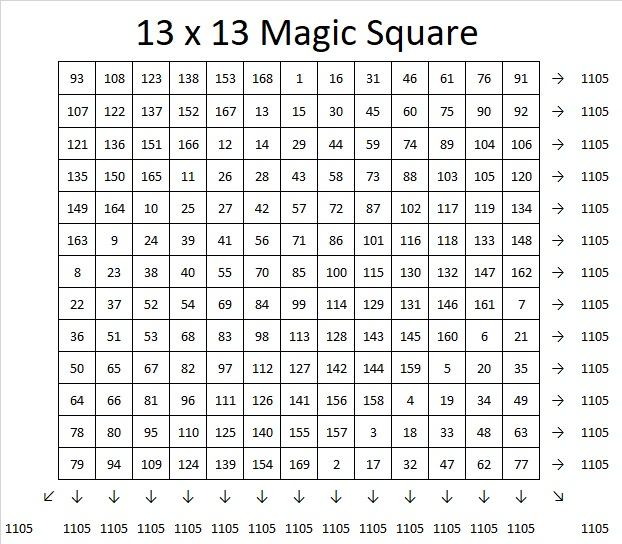If you follow the location of the numbers 1, 2, 3, 4, all the way to 169 in the magic square, you will see the pattern that I used to make that magic square. If you click on 10-factors-1102-1110  and go to the magic squares tab, you can use the same pattern or try another to create an 11 × 11, 13 × 13, or 15 × 15 magic square. The sums on the rows, columns, and diagonals will automatically populate as you write in the numbers so you can verify that you have indeed created a magic square.

1105 tiny squares can be made into a decagon so we say it is a decagonal number: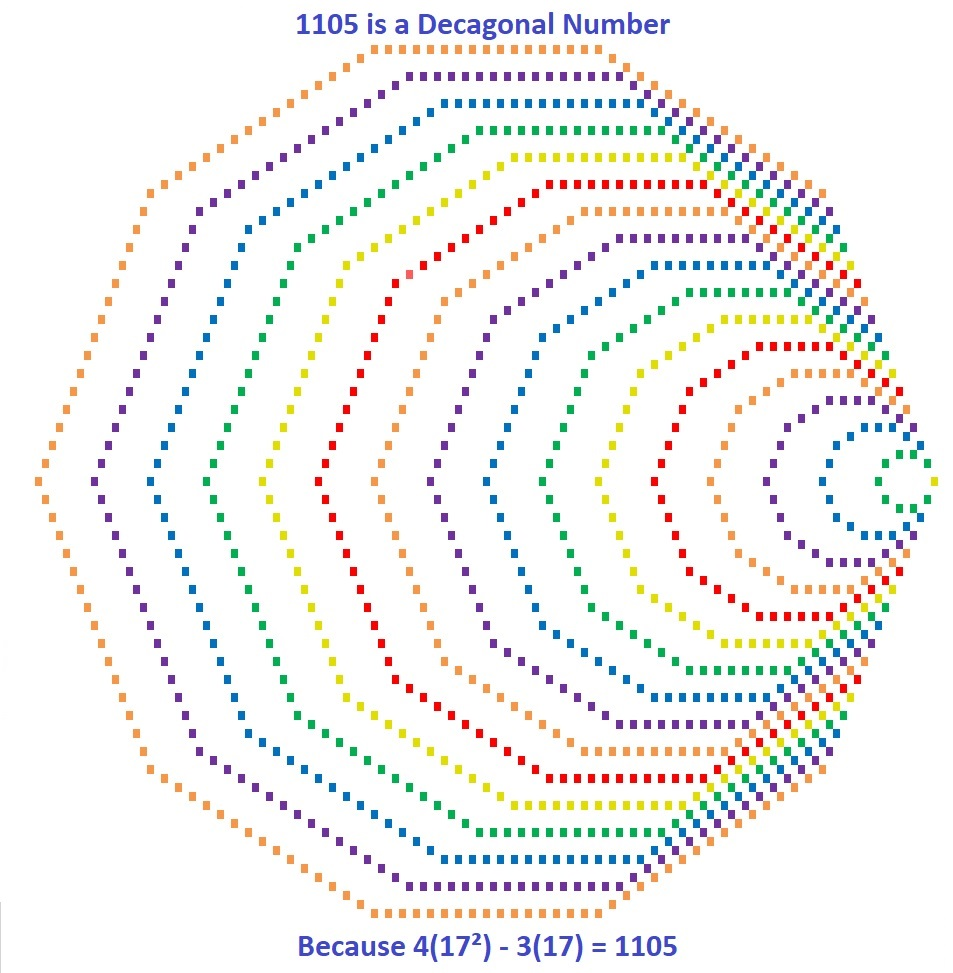Those 1105  tiny squares can also be arranged into a centered square: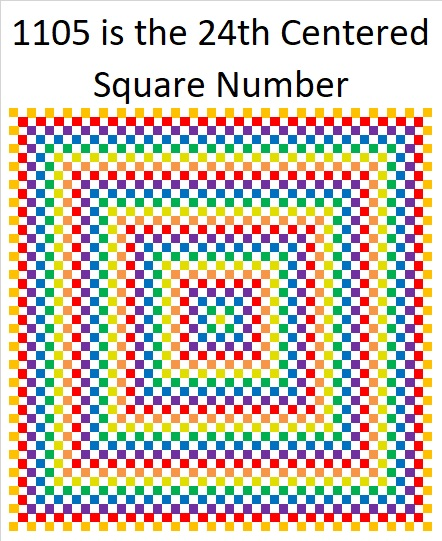Why is 1105 the 24th Centered Square Number? Because it is the sum of consecutive square numbers:
24² + 23² = 1105

But that’s not all! 1105 is the smallest number that is the sum of two squares FOUR different ways:

24² + 23² = 1105
31² + 12² = 1105
32² + 9² = 1105
33² + 4² = 1105

1105 is also the smallest number that is the hypotenuse of THIRTEEN different Pythagorean triples. Yes, THIRTEEN! (Seven was the most any previous number has had.) It is also the smallest number to have FOUR of its Pythagorean triplets be primitives (Those four are in blue type.):

47-1104-1105 calculated from 24² – 23², 2(24)(23), 24² + 23²
105-1100-1105 which is 5 times (21-220-221)
169-1092-1105 which is 13 times (13-84-85)
264-1073-1105 calculated from 2(33)(4), 33² – 4², 33² + 4²
272-1071-1105 which is 17 times (16-63-65)
425-1020-1105 which is (5-12-13) times 85
468-1001-1105 which is 13 times (36-77-85)
520-975-1105 which is (8-15-17) times 65
561-952-1105 which is 17 times (33-56-85)
576-943-1105 calculated from 2(32)(9), 32² – 9², 32² + 9²
663-884-1105 which is (3-4-5) times 221
700-855-1105 which is 5 times (140-171-221)
744-817-1105 calculated from 2(31)(12), 31² – 12², 31² + 12²

Why is it the hypotenuse more often than any previous number? Because of its factors! 1105 = 5 × 13 × 17, so it is the smallest number that is the product of THREE different Pythagorean hypotenuses.

It gets 1 triple for each of its three individual factors: 5, 13, 17, 2 triples for each of the three ways the factors can pair up with each other: 65, 85, 221, and four primitive triples for the one way they can all three be together: 1105. Thus it gets 2º×3 + 2¹×3 + 2²×1 = 3 + 6 + 4 = 13 triples.

Speaking of factors, let’s take a look at 1105’s factoring information:

• 1105 is a composite number.
• Prime factorization: 1105 = 5 × 13 × 17
• The exponents in the prime factorization are 1, 1, and 1. Adding one to each and multiplying we get (1 + 1)(1 + 1)(1 + 1) = 2 × 2 × 2 = 8. Therefore 1105 has exactly 8 factors.
• Factors of 1105: 1, 5, 13, 17, 65, 85, 221, 1105
• Factor pairs: 1105 = 1 × 1105, 5 × 221, 13 × 85, or 17 × 65
• 1105 has no square factors that allow its square root to be simplified. √1105 ≈ 33.24154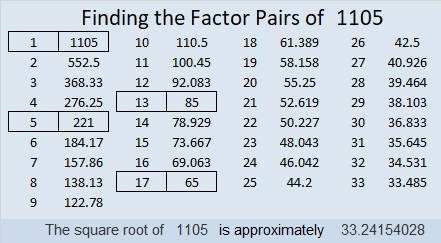1105 is also a palindrome in four different bases, and I also like the way it looks in base 8:
It’s 10001010001 in BASE 2 because 2¹º + 2⁶ + 2⁴ + 2º = 1105,
101101 in BASE 4 because 4⁵ + 4³ + 4² + 4º = 1105,
2121 in BASE 8 because 2(8³) + 1(8²) + 2(8) + 1(1) = 1105,
313 in BASE 19 because 3(19²) + 1(19) + 3(1) = 1105
1M1 in BASE 24 (M is 22 base 10) because 24² + 22(24) + 1 = 1105

Last, but certainly not least, you wouldn’t think 1105 is a prime number, but it is a pseudoprime: the second smallest Carmichael number. Only Carmichael number 561 is smaller than it is.

A Carmichael number is a composite number that behaves like a prime number by giving a false positive to all of certain quick prime number tests:
1105 passes the test p¹¹⁰⁵ Mod 1105 = p for all prime numbers p < 1105. Here is an image of my computer calculator showing 1105 passing the first five tests! Only a prime number should pass all these tests.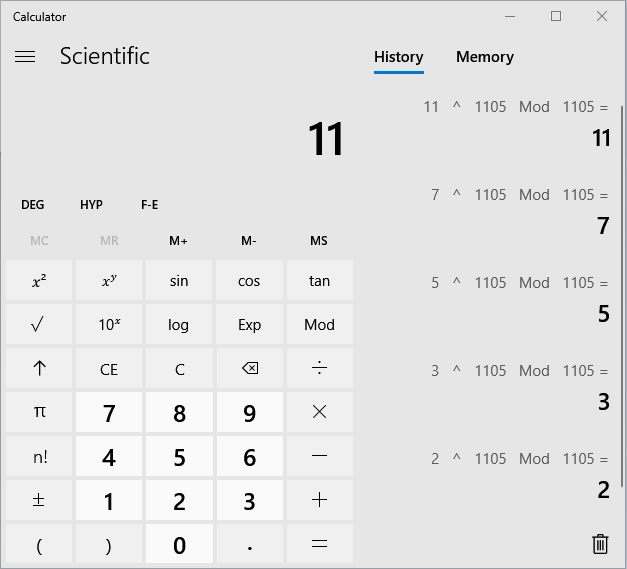1105 is indeed a number with amazing mathemagical properties!

This site uses Akismet to reduce spam. Learn how your comment data is processed.